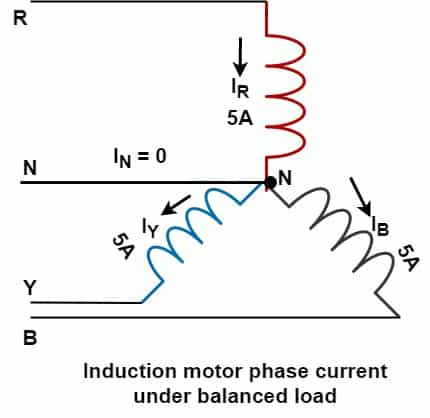# Why do 3 phase induction motors not need a neutral?

3 Phase induction motor is a balanced three-phase load. Therefore, the induction motor draws a balanced current if the supply voltage and the impedance of the motor are balance. The balance current in all three phases of the motor leads to no current in the neutral. Therefore, there is no need for neutral in the 3 phase induction motor. And, the neutral point of the star-connected motor has no connection with the ground point.

## Do 3-phase motors require a neutral?

An Induction motor has an equal impedance of all three stator winding. The winding draw current is equal in magnitude and exactly lag with their respective phase voltages. and thus the current in each phase is 120 degrees electrical apart.

Thus, the 03 phase induction motor draws a balanced current, and the vector sum of all three-phase current is zero.When the phase current of each phase is equal in magnitude, and phase currents are 120 degrees electrical apart, the phasor sum of all three-phase current is zero. Whatever, imbalance phase current flow through the neutral. In the case of 3 phase induction motor, the neutral current is zero. This is the reason why the induction motor star point is not grounded.Under the above condition, no current at any instant will flow in a neutral conductor. To understand it let’s take the three-phase waveform and instantaneous current at any instant.At point A when R phase has a maximum positive current value of 1.5 amperes. At the instant of point A, the current in phase Y  and Phase B  are both negative but equal to 0.75 amperes, each phase thus giving an exact balance of 1.5A towards the load and 1.5 A returning to the supply. If we observe the waveform and find out the instantaneous phase current value, then we will find that all the phases carry equal current.

From above it is clear that when current flows through one of the phases, then the other two phases behave as a return path and no current flows through the neutral. Therefore, there is no need for neural.  Also, the impedance of each winding is the same, therefore no current flows through the neutral.

The motor draws balance current if;

1. The supply voltage is balance
2. The impedance of winding is balance

What happens if there is an imbalance in supply voltage or impedance of the motor winding? Under these conditions, phase current does not balance, and the voltage develops at the neutral point. If we connect neutral to earth point, the current starts flowing from the neutral point to earth point. In this condition, shall we earth the neutral point of the stator? No- it is not recommended to earth the neutral point of the motor to earth. If we implement this scheme in industries for all the motors installed in a particular electrical network, then unbalance current may find paths through other motors as well. In this case, the healthy motor may trip and it becomes very difficult to find the real fault location.

To eliminate this problem, a motor protection relay is installed for motor protection. The relay trips the motor indicating negative phase sequence current (I2) fault.

Related Posts

### 3 thoughts on “Why do 3 phase induction motors not need a neutral?”

1.In my power plant negative sequence istalled at the Generator.only.
for induction motor (big motor) in not istalled.

•2.